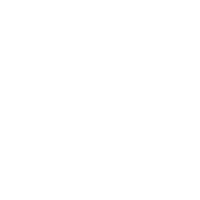# SQL in Java 8: ResultSet Streams.

With Java 8, writing SQL will change fundamentally, no matter what API you're using.

## How we write SQL in Java 7 (using JDBC)

```List<Schema> result = new ArrayList<>();
try (Connection c = getConnection()) {
String sql = "select schema_name, is_default " +
"from information_schema.schemata " +
"order by schema_name";

try (PreparedStatement stmt = c.prepareStatement(sql);
ResultSet rs = stmt.executeQuery()) {

while (rs.next()) {
System.out.println(
new Schema(rs.getString("SCHEMA_NAME"),
rs.getBoolean("IS_DEFAULT"))
);
}
}
}
```

## How we write SQL in Java 8 (using jOOλ)```try (Connection c = getConnection()) {
String sql = "select schema_name, is_default " +
"from information_schema.schemata " +
"order by schema_name";

try (PreparedStatement stmt = c.prepareStatement(sql) {

// We can wrap a Statement or a ResultSet in a
// Java 8 ResultSet Stream
SQL.stream(stmt, Unchecked.function(rs ->
new Schema(
rs.getString("SCHEMA_NAME"),
rs.getBoolean("IS_DEFAULT")
)
))
.forEach(System.out::println);
}
}
```

## How we write SQL in Java 8 (using jOOQ)```try (Connection c = getConnection()) {
String sql = "select schema_name, is_default " +
"from information_schema.schemata " +
"order by schema_name";

DSL.using(c)
.fetch(sql)

// We can use lambda expressions to map jOOQ Records
.map(rs -> new Schema(
rs.getValue("SCHEMA_NAME", String.class),
rs.getValue("IS_DEFAULT", boolean.class)
))

// ... and then profit from the new Collection methods
.forEach(System.out::println);
}
```

## How we write SQL in Java 8 (using Spring JDBC)

```try (Connection c = getConnection()) {
String sql = "select schema_name, is_default " +
"from information_schema.schemata " +
"order by schema_name";

new JdbcTemplate(
new SingleConnectionDataSource(c, true))

// We can use lambda expressions as RowMappers
.query(sql, (rs, rowNum) ->
new Schema(
rs.getString("SCHEMA_NAME"),
rs.getBoolean("IS_DEFAULT")
))

// ... and then profit from the new Collection methods
.forEach(System.out::println);
}
```

## How we write SQL in Java 8 (using Apache DbUtils)

```try (Connection c = getConnection()) {
String sql = "select schema_name, is_default " +
"from information_schema.schemata " +
"order by schema_name";

new QueryRunner()
.query(c, sql, new ArrayListHandler())

// We can transform any Collection into a Stream
.stream()
.map(array -> new Schema(
(String) array,
(Boolean) array
))

// ... and then profit from the new Stream methods
.forEach(System.out::println);
}
```

## With Java 8, writing SQL will finally be fun again!